# Scale Drawings and Scale Models Learn to understand

• Slides: 9Scale Drawings and Scale Models Learn to understand ratios and proportions in scale drawings. Learn to use ratios and proportions with scale.Scale Drawings and Scale Models Vocabulary scale drawing scale factor scale model scale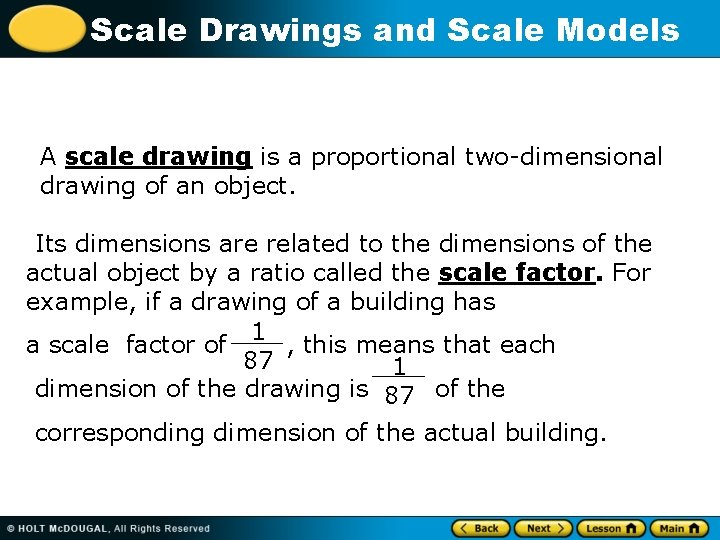Scale Drawings and Scale Models A scale drawing is a proportional two-dimensional drawing of an object. Its dimensions are related to the dimensions of the actual object by a ratio called the scale factor. For example, if a drawing of a building has 1 a scale factor of , this means that each 87 1 dimension of the drawing is 87 of the corresponding dimension of the actual building.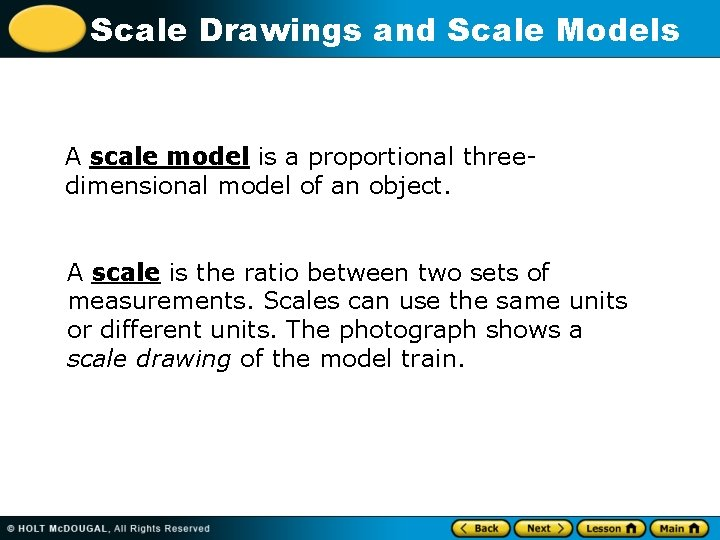Scale Drawings and Scale Models A scale model is a proportional threedimensional model of an object. A scale is the ratio between two sets of measurements. Scales can use the same units or different units. The photograph shows a scale drawing of the model train.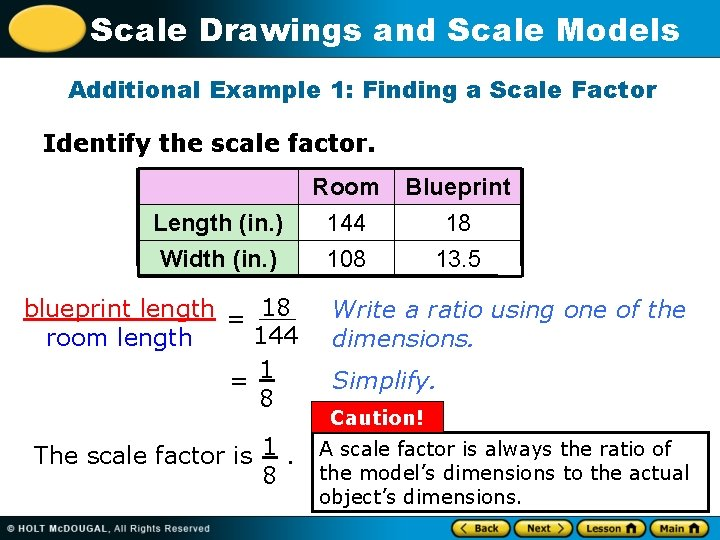Scale Drawings and Scale Models Additional Example 1: Finding a Scale Factor Identify the scale factor. Length (in. ) Width (in. ) blueprint length = 18 144 room length = 1 8 The scale factor is 1. 8 Room 144 108 Blueprint 18 13. 5 Write a ratio using one of the dimensions. Simplify. Caution! A scale factor is always the ratio of the model’s dimensions to the actual object’s dimensions.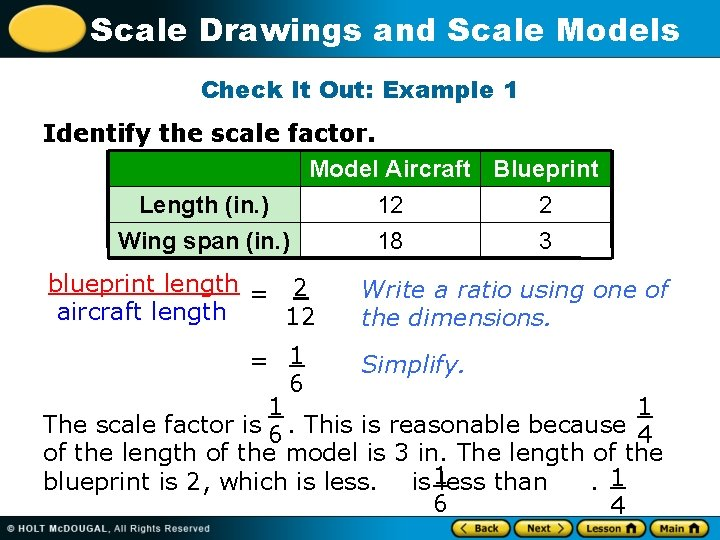Scale Drawings and Scale Models Check It Out: Example 1 Identify the scale factor. Model Aircraft Blueprint Length (in. ) 12 2 Wing span (in. ) 18 3 blueprint length = 2 aircraft length 12 Write a ratio using one of the dimensions. = 1 Simplify. 6 1 1 The scale factor is 6. This is reasonable because 4 of the length of the model is 3 in. The length of the blueprint is 2, which is less. is 1 less than. 1 6 4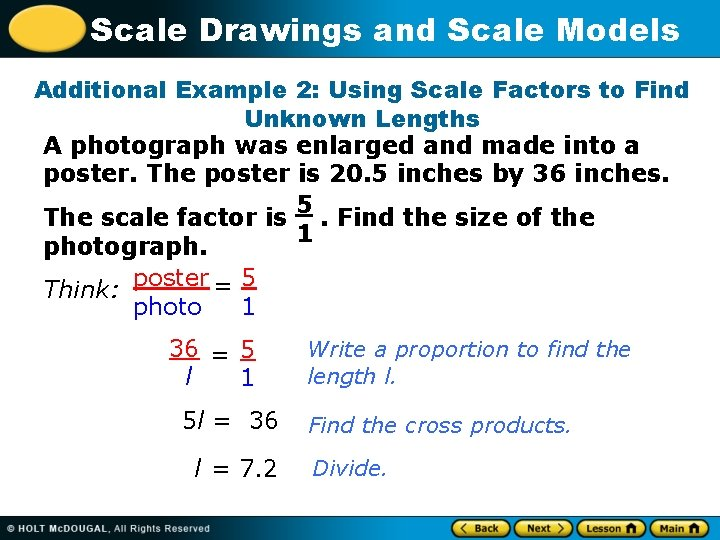Scale Drawings and Scale Models Additional Example 2: Using Scale Factors to Find Unknown Lengths A photograph was enlarged and made into a poster. The poster is 20. 5 inches by 36 inches. 5 The scale factor is. Find the size of the 1 photograph. poster = 5 Think: photo 1 36 = 5 l 1 5 l = 36 l = 7. 2 Write a proportion to find the length l. Find the cross products. Divide.Scale Drawings and Scale Models Additional Example 2 Continued A photograph was enlarged and made into a poster. The poster is 20. 5 inches by 36 inches. The scale factor is 5. Find the size of the 1 photograph. Think: poster = 5 photo 1 20. 5 = 5 w 1 5 w = 20. 5 w = 4. 1 Write a proportion to find the width w. Find the cross products. Divide. The photo is 7. 2 in. long and 4. 1 in. wide.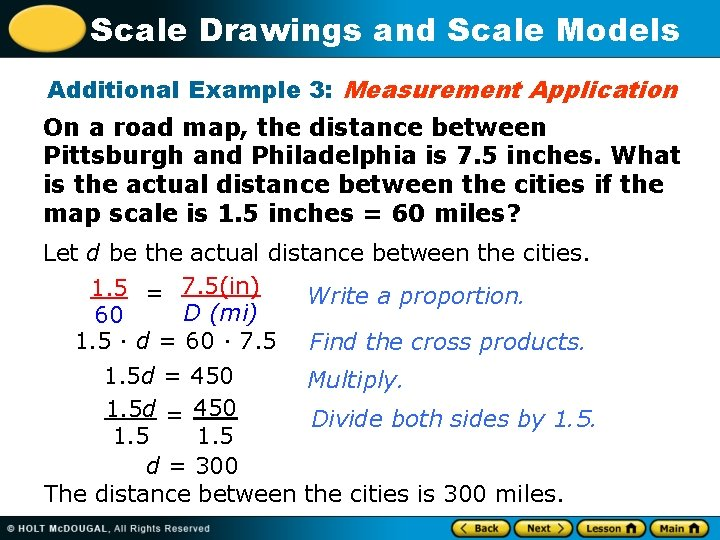Scale Drawings and Scale Models Additional Example 3: Measurement Application On a road map, the distance between Pittsburgh and Philadelphia is 7. 5 inches. What is the actual distance between the cities if the map scale is 1. 5 inches = 60 miles? Let d be the actual distance between the cities. 1. 5 = 7. 5(in) Write a proportion. D (mi) 60 1. 5 · d = 60 · 7. 5 Find the cross products. 1. 5 d = 450 Multiply. 1. 5 d = 450 Divide both sides by 1. 5 d = 300 The distance between the cities is 300 miles.